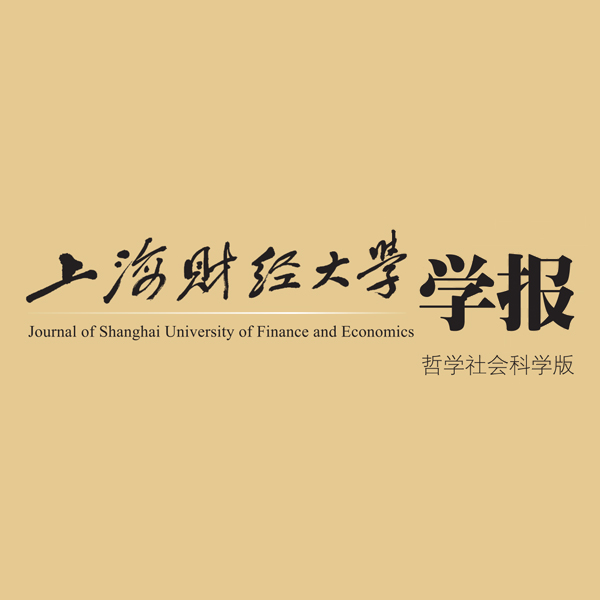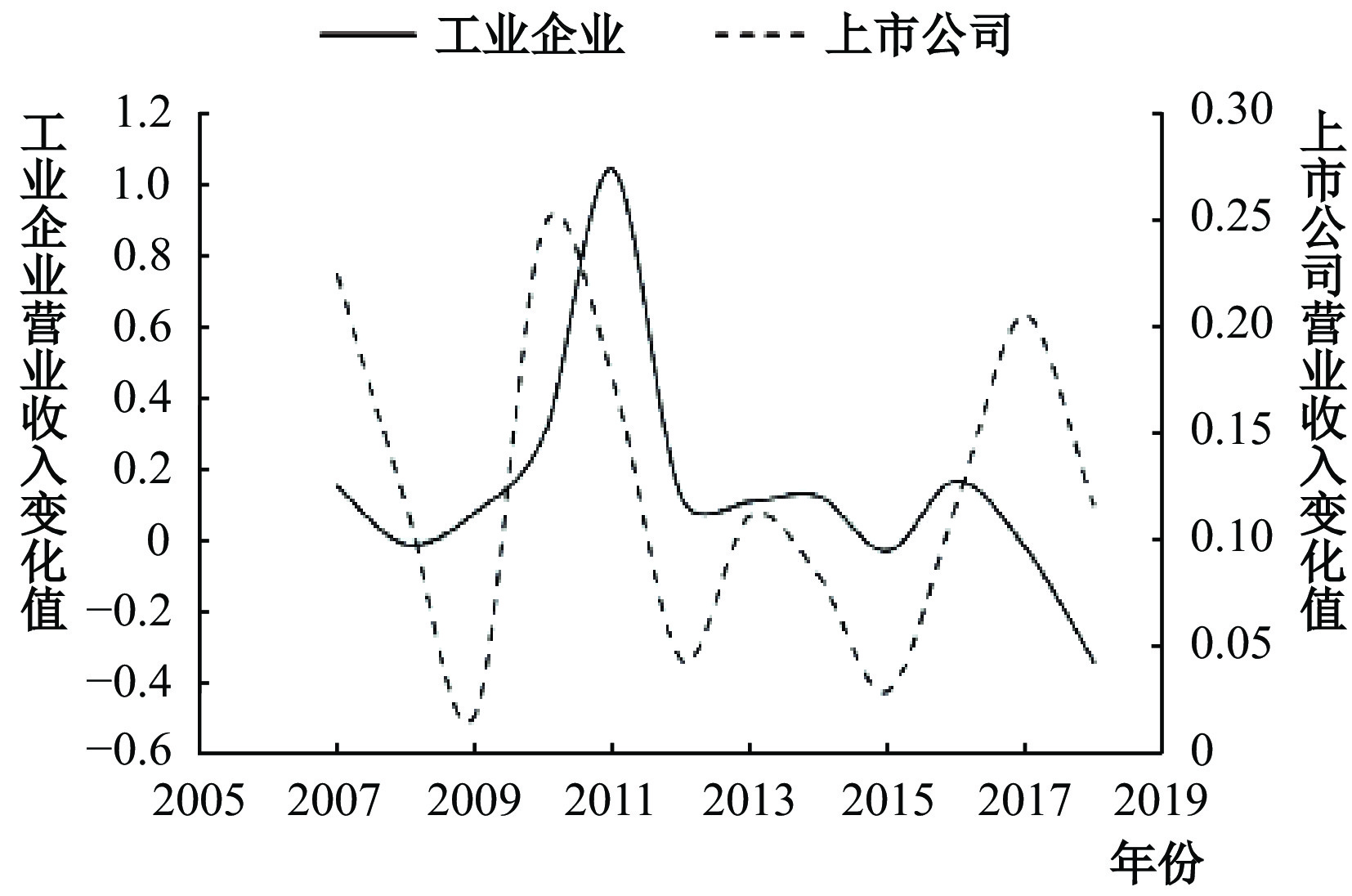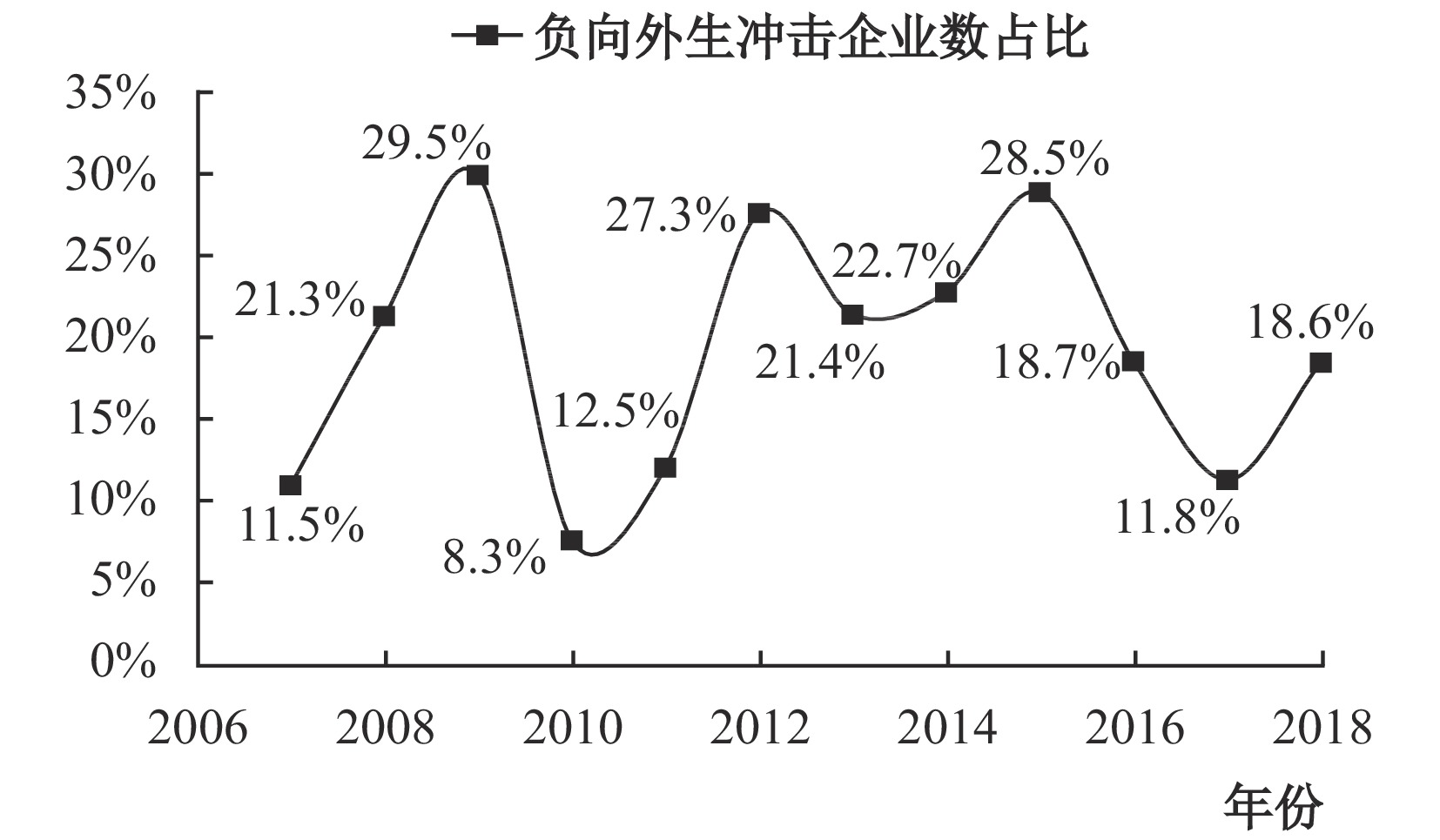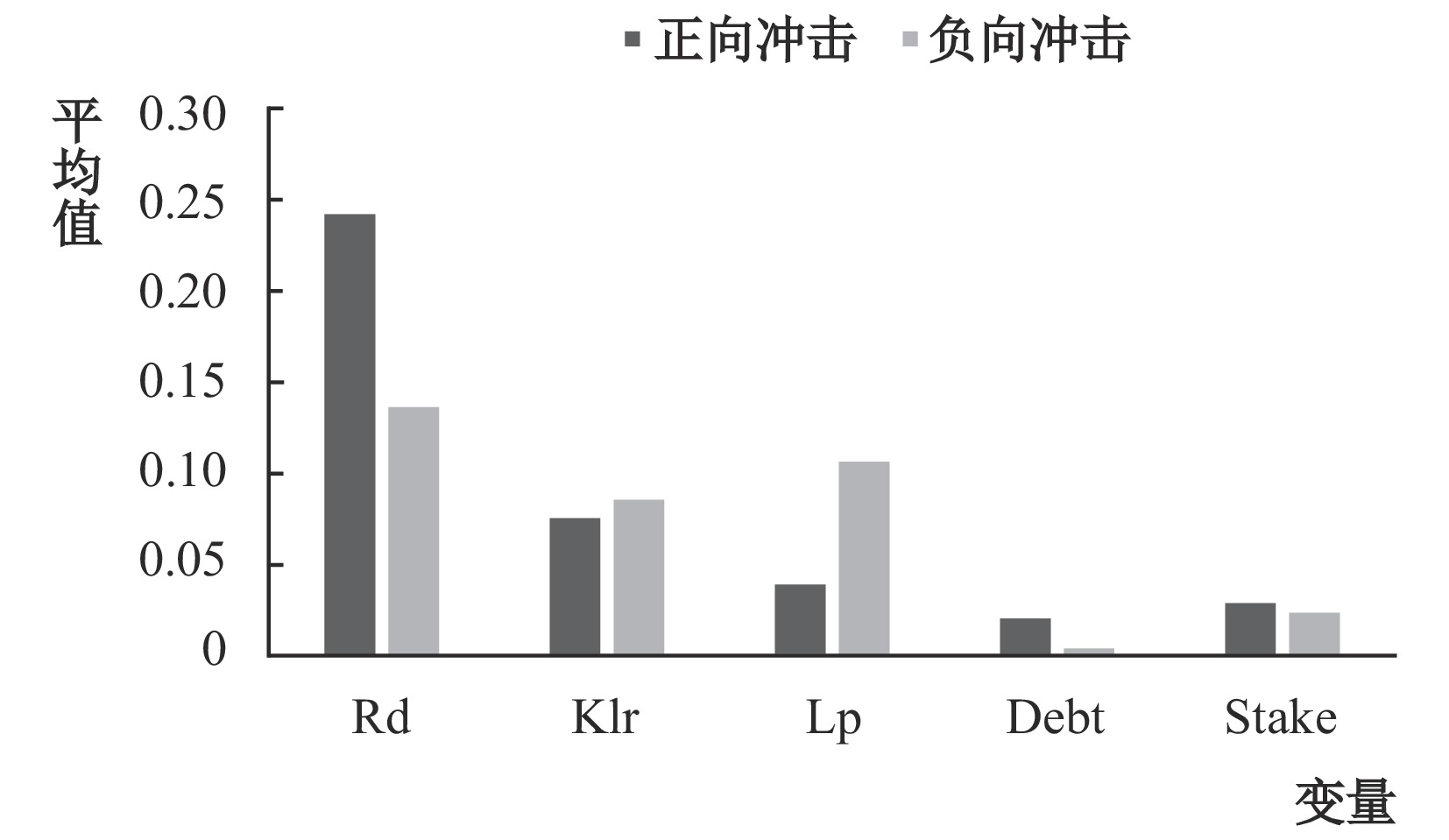﻿ 外生冲击、融资模式选择与制造业升级《上海财经大学学报》
2020第22卷第4期

1.1.吉林大学 数量经济研究中心，吉林 长春 130012;
2.2.吉林大学 商学院，吉林 长春 130012图 1 制造业企业营业收入变化趋势图

H1a：总体上，债权融资对中国制造业升级具有促进作用。

H1b：总体上，债权融资对中国制造业升级具有抑制作用。

H1c：总体上，债权融资对中国制造业升级不存在显著影响。

H2：总体上，股权融资对中国制造业升级具有促进作用。

(二) 外生冲击对融资模式与制造业升级关系的影响机制分析

H3：与正向外生冲击相比，负向外生冲击下债权融资对制造业升级具有更严重的抑制作用，或具有更不明显的促进作用。

H4：与正向外生冲击相比，负向外生冲击下股权融资对制造业升级具有更加明显的促进作用。

(二) 产业升级的测度

(三) 模型设计

 $R{d_{i,t}} = a + {\alpha _1}Deb{t_{i,t}} + \sum\limits_{1 \leqslant i} {{\beta _i}control{s_{i,t}}} + {\varepsilon _{i,t}}$ (1)
 $R{d_{i,t}} = a + {\alpha _1}Deb{t_{i,t}} + {\alpha _2} \times I \times Deb{t_{i,t}} + \sum\limits_{1 \leqslant i} {{\beta _i}control{s_{i,t}}} + {\varepsilon _{i,t}}$ (2)
 $R{d_{i,t}} = a + {\alpha _1}S\!tak{e_{i,t}} + \sum\limits_{1 \leqslant i} {{\beta _i}control{s_{i,t}}} + {\varepsilon _{i,t}}$ (3)
 $R{d_{i,t}} = a + {\alpha _1}S\!tak{e_{i,t}} + {\alpha _2} \times I \times S\!tak{e_{i,t}} + \sum\limits_{1 \leqslant i} {{\beta _i}control{s_{i,t}}} + {\varepsilon _{i,t}}$ (4)
 $Kl{r_{i,t}} = a + {\alpha _1}Deb{t_{i,t}} + \sum\limits_{1 \leqslant i} {{\beta _i}control{s_{i,t}}} + {\varepsilon _{i,t}}$ (5)
 $Kl{r_{i,t}} = a + {\alpha _1}Deb{t_{i,t}} + {\alpha _2} \times I \times Deb{t_{i,t}} + \sum\limits_{1 \leqslant i} {{\beta _i}control{s_{i,t}}} + {\varepsilon _{i,t}}$ (6)
 $Kl{r_{i,t}} = a + {\alpha _1}S\!tak{e_{i,t}} + \sum\limits_{1 \leqslant i} {{\beta _i}control{s_{i,t}}} + {\varepsilon _{i,t}}$ (7)
 $Kl{r_{i,t}} = a + {\alpha _1}S\!tak{e_{i,t}} + {\alpha _2} \times I \times S\!tak{e_{i,t}} + \sum\limits_{1 \leqslant i} {{\beta _i}control{s_{i,t}}} + {\varepsilon _{i,t}}$ (8)

$Rd$

$Klr$

$I$

$Debt$

$S\!take$

$Controls$

 $L{p_{i,t}} = a + {\alpha _1}Deb{t_{i,t}} + \sum\limits_{1 \leqslant i} {{\beta _i}control{s_{i,t}}} + {\varepsilon _{i,t}}$ (9)
 $L{p_{i,t}} = a + {\alpha _1}Deb{t_{i,t}} + {\alpha _2} \times I \times Deb{t_{i,t}} + \sum\limits_{1 \leqslant i} {{\beta _i}control{s_{i,t}}} + {\varepsilon _{i,t}}$ (10)
 $L{p_{i,t}} = a + {\alpha _1}S\!tak{e_{i,t}} + \sum\limits_{1 \leqslant i} {{\beta _i}control{s_{i,t}}} + {\varepsilon _{i,t}}$ (11)
 $L{p_{i,t}} = a + {\alpha _1}S\!tak{e_{i,t}} + {\alpha _2} \times I \times S\!tak{e_{i,t}} + \sum\limits_{1 \leqslant i} {{\beta _i}control{s_{i,t}}} + {\varepsilon _{i,t}}$ (12)

$Lp$

 变量类型 变量名称 变量符号 测量方式 依据 被解释变量 研发支出 Rd 研发支出变化率 李汇东等（2013）、胡亚茹等（2018） 资本劳动比 Klr 资本劳动比变化率 张杰等（2016） 劳动生产率 Lp 劳动生产率变化值 李永友和严岑（2018） 解释变量 债权融资 Debt （银行贷款+应付债券）变化额/总资产 韩珣等（2017） 股权融资 Stake （股本+资本公积）变化额/期初营业收入 李汇东等（2013） 调节变量 外生冲击 I I=1，正向冲击；I1=0，其他 Aghion等（2012）、孙巍和 董文宇（2019） 控制变量 收入增长率 Growth 营业收入变化额/期初营业收入 胡亚茹等（2018） 劳动力价格 Wage （职工薪酬支出+应付职工薪酬本期 增加额）/员工人数，取对数 李永友和严岑（2018） 财务杠杆 Lev 总负债/总资产 陶锋等（2017） 竞争程度 Compt 销售费用 /营业收入 李汇东等（2013） 固定资产比率 Fixr 固定资产/总资产 陶锋等（2017） 注：所涉及固定资产以及增加值变量均经过价格平减处理。
(四) 数据及相关描述性统计图 2 负向外生冲击企业数目占比变化趋势图图 3 外生冲击下的产业升级和融资模式

 变量 样本数 均值 标准差 极小值 极大值 $Rd$ 10937 0.221 0.364 −0.312 1.185 $Klr$ 17539 0.086 0.309 −0.338 0.958 $Lp$ 14106 0.056 0.322 −0.633 0.781 $Debt$ 17544 0.016 0.067 −0.115 0.158 $S\!take$ 17544 0.029 0.068 −0.008 0.249 $Growth$ 17546 0.155 0.256 −0.261 0.772 $Wage$ 17533 10.971 0.479 10.027 11.859 $Lev$ 19832 0.419 0.197 0.102 0.771 $Compt$ 19834 7.072 6.574 0.795 25.805 $Fixr$ 19832 0.245 0.138 0.051 0.537

 Rd Rd Rd Rd Klr Klr Klr Klr Debt−主效应 −0.240***（−2.95） 0.305***（7.21） Debt−正向冲击 −0.225***（−2.72） 0.308***（6.55） Debt−负向冲击 −0.274***（−3.05） 0.297***（4.29） Stake−主效应 0.194***（3.05） 0.152***（3.42） Stake−正向冲击 0.179***（2.62） 0.111**（2.38） Stake−负向冲击 0.243*（1.77） 0.260***（3.09） N 7878 7878 7878 7878 13306 13306 13306 13306 R2 0.151 0.151 0.151 0.151 0.477 0.477 0.474 0.475 注：*、**、***分别表示10%、5%、1%的显著性水平，括号内为Z或T检验值；为节省篇幅，表中省略了控制变量的系数，回归已经控制固定效应、年度效应以及行业效应，主要解释变量系数通过调节变量法计算得出，已通过联合系数显著性检验。表4、表5、表6同。

 Lp Lp Lp Lp Debt−主效应 −0.144***（−3.08） Debt−正向冲击 −0.122**（−2.37） Debt−负向冲击 −0.202**（−2.15） Stake−主效应 0.006***（3.46） Stake−正向冲击 0.004**（2.02） Stake−负向冲击 0.011***（3.39） N 14084 14084 14079 14079 R2 0.431 0.342 0.341 0.342

(二) 稳健性检验

 $Rd$ $Rd$ $Klr$ $Klr$ $Lp$ $Lp$ $Debt$ -正向冲击 −1.417***（−4.15） 1.006***（2.64） −0.816**（−2.16） $Debt$ -负向冲击 −2.437***（−6.12） 0.955*（1.79） −0.150*（−1.85） $S\!take$ -正向冲击 1.369***（3.56） 0.057（1.06） 0.016（1.14） $S\!take$ -负向冲击 1.722**（2.04） 1.046*（1.87） 0.035（1.41） $N$ 7879 7879 13300 13300 11979 11975 $Wald$ 1175.28 1182.77 1296.81 1293.25 1567.34 1562.50
(三) 基于所有制形式的异质性分析图 4 不同所有制企业融资模式与产业升级的特征差异

 升级过程 升级结果 $Rd$ -国企 $Rd$ -非国企 $Klr$ -国企 $Klr$ -非国企 $Lp$ -国企 $Lp$ -非国企 $Debt$ -正向冲击 -0.152（-0.82） -0.231**（-2.49） 0.262***（3.99） 0.285***（4.20） -0.176**（-2.13） -0.068（-1.05） $Debt$ -负向冲击 -0.236（-1.28） -0.313***（-2.89） 0.323***（3.20） 0.227***（2.57） -0.317**（-2.21） -0.055（-0.44） $S\!take$ -正向冲击 0.303（1.79） 0.162**（2.19） 0.036（0.43） 0.128**（2.24） 0.008**（2.19） 0.001（0.35） $S\!take$ -负向冲击 -0.236（-0.93） 0.432***（2.61） 0.616***（4.01） 0.094（1.01） 0.017***（3.18） 0.005（1.21） $N$ 2428 5450 5679 7627 5650 8429 ${R^2}$ 0.157 0.161 0.430 0.530 0.349 0.355

  安苑, 王珺. 地方政府财政行为周期性、产业成长与结构失衡——基于产业外部融资依赖度的分析[J].财经研究,2014(11).  程新生, 谭有超, 刘建梅. 非财务信息、外部融资与投资效率——基于外部制度约束的研究[J].管理世界,2012(7).  戴翔, 张雨. 开放条件下我国本土企业升级能力的影响因素研究——基于昆山制造业企业问卷的分析[J].经济学（季刊）,2013(4).  冯根福. 双重委托代理理论: 上市公司治理的另一种分析框架——兼论进一步完善中国上市公司治理的新思路[J].经济研究,2004(12).  韩鹏, 岳园园. 企业创新行为信息披露的经济后果研究——来自创业板的经验证据[J].会计研究,2016(1).  韩珣, 田光宁, 李建军. 非金融企业影子银行化与融资结构——中国上市公司的经验证据[J].国际金融研究,2017(10).  胡亚茹, 陈丹丹, 刘震. 融资约束、企业研发投入的周期性与平滑机制——基于企业所有制视角[J].产业经济研究,2018(2).  黄宏斌, 翟淑萍, 陈静楠. 企业生命周期、融资方式与融资约束——基于投资者情绪调节效应的研究[J].金融研究,2016(7).  李广众, 叶敏健, 郑颖. 资本结构与员工劳动生产率[J].管理科学学报,2018(2).  李汇东, 唐跃军, 左晶晶. 用自己的钱还是用别人的钱创新? ——基于中国上市公司融资结构与公司创新的研究[J].金融研究,2013(2).  李林木, 汪冲. 税费负担、创新能力与企业升级——来自“新三板”挂牌公司的经验证据[J]. 经济研究, 2017, （11）. http://www.cnki.com.cn/Article/CJFDTOTAL-JJYJ201711018.htm  李永友, 严岑. 服务业“营改增”能带动制造业升级吗?[J].经济研究,2018(4).  刘小玄, 周晓艳. 金融资源与实体经济之间配置关系的检验——兼论经济结构失衡的原因[J]. 金融研究, 2011, （2）. http://www.cqvip.com/QK/71588X/201210/44224531.html  刘志东, 高洪玮. 中国制造业出口对美国企业创新的影响[J]. 中国工业经济, 2019, （8）. http://www.cnki.com.cn/Article/CJFDTotal-GGYY201908010.htm  吕劲松. 关于中小企业融资难、融资贵问题的思考[J]. 金融研究, 2015, （11）. http://www.cnki.com.cn/Article/CJFDTotal-ZGSM201819017.htm  马光荣, 刘明, 杨恩艳. 银行授信、信贷紧缩与企业研发[J].金融研究,2014(7).  马红, 侯贵生. 混合所有制改革、地方国企依赖与国有企业创新升级——基于制造业的实证研究[J].上海财经大学学报,2019(2).  孙巍, 董文宇. 市场冲击与企业投资的创新效应研究——兼论去杠杆和贸易战对“中国创造”进程的潜在影响[J].中大财经大学学报,2019(8).  孙巍, 宋南. 投资与研发行为的外生性市场需求扩张理论及其实证[J].产业经济评论(山东大学),2019(2).  孙玮, 王九云, 成力为. 技术来源与高技术产业创新生产率——基于典型相关分析的中国数据实证研究[J]. 科学学研究, 2010, （7）. http://www.cnki.com.cn/Article/CJFDTotal-KXYJ201007019.htm  陶锋, 胡军, 李诗田, 等. 金融地理结构如何影响企业生产率? ——兼论金融供给侧结构性改革[J].经济研究,2017(9).  王桂军, 卢潇潇. “一带一路”倡议与中国企业升级[J]. 中国工业经济, 2019, （3）. http://www.cnki.com.cn/Article/CJFDTotal-GGYY201903006.htm  王旭, 褚旭. 中国制造业绿色技术创新与融资契约选择[J].科学学研究,2019(2).  王玉泽, 罗能生, 刘文彬. 什么样的杠杆率有利于企业创新[J]. 中国工业经济, 2019, （3）. http://www.cnki.com.cn/Article/CJFDTotal-GGYY201903011.htm  温军, 冯根福, 刘志勇. 异质债务、企业规模与R&D投入[J]. 金融研究, 2011, （1）. http://www.cnki.com.cn/Article/CJFDTotal-JRYJ201101014.htm  夏冠军, 陆根尧. 资本市场促进了高新技术企业研发投入吗——基于中国上市公司动态面板数据的证据[J].科学学研究,2012(9).  杨贵军, 周亚梦, 孙玲莉. 基于Benford-Logistic模型的企业财务风险预警方法[J].数量经济技术经济研究,2019(10).  于震, 王肖梦, 刘淼. 中国上市企业融资约束成因研究[J].数量经济研究,2019(2).  张杰, 郑文平, 翟福昕. 融资约束影响企业资本劳动比吗? ——中国的经验证据[J].经济学（季刊）,2016(3).  张璇, 刘贝贝, 汪婷, 等. 信贷寻租、融资约束与企业创新[J]. 经济研究, 2017, （5）. http://www.cnki.com.cn/Article/CJFDTOTAL-JJYJ201705020.htm  张一林, 龚强, 荣昭. 技术创新、股权融资与金融结构转型[J].管理世界,2016(11).  赵进文, 丁林涛. 贸易开放度、外部冲击与通货膨胀: 基于非线性STR模型的分析[J].世界经济,2012(9).  Aghion P, Askenazy P, Berman N, et al. Credit constraints and the cyclicality of R&D investment: Evidence from France[J].Journal of the European Economic Association,2012,10(5):1001–1024.  Allen F, Bartiloro L, Gu X, et al. Does economic structure determine financial structure?[J].Journal of International Economics,2018,114:389–409.  Bartoloni E. Capital structure and innovation: Causality and determinants[J].Empirica,2013,40(1):111–151.  Benfratello L, Schiantarelli F, Sembenelli A. Banks and innovation: Microeconometric evidence on Italian firms[J]. Journal of Financial Economics, 2008, 90（2）: 197–217. http://www.sciencedirect.com/science/article/pii/S0304405X08001293  Brown J R, Martinsson G, Petersen B C. Law, stock markets, and innovation[J].The Journal of Finance,2013,68(4):1517–1549.  Butler A W, Cornaggia J. Does access to external finance improve productivity? Evidence from a natural experiment[J]. Journal of Financial Economics, 2011, 99（1）: 184–203. http://public-prod-acquia.gsb.stanford.edu/sites/default/files/documents/fin_02_09_cornaggia.pdf  Carpenter R E, Petersen B C. Capital market imperfections, high-tech investment, and new equity financing[J].The Economic Journal,2002,112(477):F54–F72.  Daniel K, Hirshleifer D, Subrahmanyam A. Investor psychology and security market under- and overreactions[J].Journal of Finance,1998,53(6):1839–1885.  Faulkender M, Petersen M A. Does the source of capital affect capital structure?[J].Review of Financial Studies,2006,19(1):45–79.  Gereffi G. International trade and industrial upgrading in the apparel commodity Chain[J].Journal of International Economics,1999,48(1):37–70.  He J, Tian X. The dark side of analyst coverage: The case of innovation[J].Journal of Financial Economics,2013,109(3):856–878.  Hsu P H, Tian X, Xu Y. Financial development and innovation: Cross-country evidence[J].Journal of Financial Economics,2014,112(1):116–135.  Humphrey J, Schmitz H. How does insertion in global value Chains affect upgrading in industrial clusters? [J]. Regional Studies, 2002, 36（9）: 1017–1027. https://www.researchgate.net/profile/Hubert_Schmitz/publication/24087684_How_Does_Insertion_in_Global_Value_Chains_Affect_Upgrading_in_Industrial_Clusters/links/57eff82b08ae280dd0aea5bc.pdf  Korajczyk R A, Levy A. Capital structure choice: Macroeconomic conditions and financial constraints[J].Journal of Financial Economics,2003,68(1):75–109.  Laeven L, Valencia F. The use of blanket guarantees in banking crises[J].Journal of International Money and Finance,2012,31(5):1220–1248.  Meyer L H. The present and future roles of banks in small business finance[J]. Journal of Banking & Finance, 1998, 22（6–8）: 1109–1116. http://www.sciencedirect.com/science/article/pii/S0378426698000193  Myers S C, Majluf N S. Corporate financing and investment decisions when firms have information that investors do not have[J]. Journal of Financial Economics, 1984, 13（2）: 187–221. https://www.zhangqiaokeyan.com/open-access_resources_thesis/0100057855452.html  Opler T C, Titman S. Financial distress and corporate performance[J].The Journal of Finance,1994,49(3):1015–1040.  Porter M E. The competitive advantage of nations[M]. New York: Free Press, 1990.  Sun W, Dong K Q, Zhao T Y. Market demand dynamic induced mechanism in China’s steel industry[J].Resources Policy,2017,51:13–21.
Exogenous Shocks, Financing Mode Selection and Manufacturing Upgrading: Also on Financial Supply-Side Reforms during Economic and Trade Frictions along with the COVID-19 Epidemic
Sun Wei1, Dong Wenyu1,2, Song Nan1,2
1.Center for Quantitative Economics，Jilin University，Jilin Changchun 130012，China;